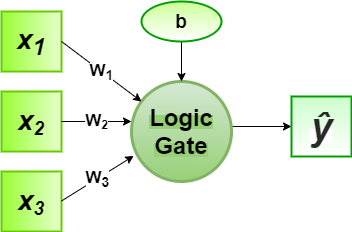# Perceptron Algorithm for Logic Gate with 3-bit Binary Input

In the field of Machine Learning, the Perceptron is a Supervised Learning Algorithm for binary classifiers. The Perceptron Model implements the following function:For a particular choice of the weight vectorand bias parameter, the model predicts outputfor the corresponding input vector.
The logical function truth table of AND, OR, NAND, NOR gates for 3-bit binary variables, i.e, the input vectorand the corresponding output0 0 0 0 0 1 1
0 0 1 0 1 1 0
0 1 0 0 1 1 0
0 1 1 0 1 1 0
1 0 0 0 1 1 0
1 0 1 0 1 1 0
1 1 0 0 1 1 0
1 1 1 1 1 0 0

Now for the corresponding weight vectorof the input vector, the associated Perceptron Function can be defined as:For the implementation, considered weight parameters areand the bias parameter isfor every logic gates-1 1 -1 -11 1 -1 -11 1 -1 -1-2 -0.9 3 1

Python Implementation:

 # importing python library  import numpy as np     # sigmoid activation function  def activationFunction(model, type ="sigmoid"):     return {         "sigmoid": 1 / (1 + np.exp(-model))     }[type]     # designing perceptron model  def perceptronModel(weights, inputs, bias):     model = np.add(np.dot(inputs, weights), bias)     logic = activationFunction(model, type ="sigmoid")     return np.round(logic)     # computation model  def compute(data, logicGate, weights, bias):     weights = np.array(weights)     output = np.array([ perceptronModel(weights,                datum, bias) for datum in data ])     return output     # Print Output  def printOutput(dataset, name, data):     print("Logic Function: {}".format(name.upper()))     print("X1\tX2\tX3\tY")     toPrint = ["{1}\t{2}\t{3}\t{0}".format(output, *datas)                  for datas, output in zip(dataset, data)]     for i in toPrint:         print(i)     # main function  def main():     # 3 bit binary data     dataset = np.array([       [0, 0, 0],       [0, 0, 1],       [0, 1, 0],       [0, 1, 1],       [1, 0, 0],       [1, 0, 1],       [1, 1, 0],       [1, 1, 1]     ])        # Parameters of every Logic Gates     # weight parameters: w1, w2, w3     # bias parameter: b     logicGate = {         "and": compute(dataset, "and", [1, 1, 1], -2),         "or": compute(dataset, "or", [1, 1, 1], -0.9),         "nand": compute(dataset, "nand", [-1, -1, -1], 3),         "nor": compute(dataset, "nor", [-1, -1, -1], 1)     }     for gate in logicGate:         printOutput(dataset, gate, logicGate[gate])     if __name__ == '__main__':     main()

Output:

Logic Function: AND
X1    X2    X3    Y
0    0    0    0.0
0    0    1    0.0
0    1    0    0.0
0    1    1    0.0
1    0    0    0.0
1    0    1    0.0
1    1    0    0.0
1    1    1    1.0
Logic Function: OR
X1    X2    X3    Y
0    0    0    0.0
0    0    1    1.0
0    1    0    1.0
0    1    1    1.0
1    0    0    1.0
1    0    1    1.0
1    1    0    1.0
1    1    1    1.0
Logic Function: NAND
X1    X2    X3    Y
0    0    0    1.0
0    0    1    1.0
0    1    0    1.0
0    1    1    1.0
1    0    0    1.0
1    0    1    1.0
1    1    0    1.0
1    1    1    0.0
Logic Function: NOR
X1    X2    X3    Y
0    0    0    1.0
0    0    1    0.0
0    1    0    0.0
0    1    1    0.0
1    0    0    0.0
1    0    1    0.0
1    1    0    0.0
1    1    1    0.0


Here, the model predicted output () for each of the test inputs are exactly matched with the AND, OR, NAND, NOR logic gates conventional output ()s according to the truth table for 3-bit binary input.
Hence, it is verified that the perceptron algorithm for all these logic gates is correctly implemented.

Attention geek! Strengthen your foundations with the Python Programming Foundation Course and learn the basics.

To begin with, your interview preparations Enhance your Data Structures concepts with the Python DS Course.

My Personal Notes arrow_drop_upCheck out this Author's contributed articles.

If you like GeeksforGeeks and would like to contribute, you can also write an article using contribute.geeksforgeeks.org or mail your article to contribute@geeksforgeeks.org. See your article appearing on the GeeksforGeeks main page and help other Geeks.

Please Improve this article if you find anything incorrect by clicking on the "Improve Article" button below.